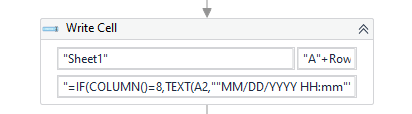# How to write add a variable to write cell formula

Dear team,

I want to write cell and with formula below, but A2, A3, A4 are variables, may I know how to add it? Thanks.

“=IF(COLUMN()=8,TEXT(A2,”“MM/DD/YYYY HH:mm”“)&”“-”“&A3&”“-”“&A4,A2&”“-”“&A3&”“-”“&A4)”are you getting any error now?

no error, but need to replace A2, A3, A4 with some variable values.

try to use “+variable name+”

get some error

Hi @li.yu ,

Could you check with the Below Formula :

``````"=IF(COLUMN()=8,TEXT(A"+Row2Po+",""MM/DD/YYYY HH:mm"")&""-""&A"+Row3Po+"&""-""&A"+Row4Po+",A"+Row2Po+"&""-""&A"+Row3Po+"&""-""&A"+Row4Po+")"
``````

Assuming that all RowPo variables are string type, this should be the formula you required.

1 Like